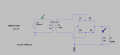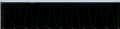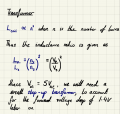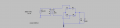# Designing an AC to DC converter

#### Sid2001

Joined Dec 7, 2016
29
Hey everyone, I'm a year 1 undergraduate Physics student taking a module in Advanced Electronics. As part of a project, I am required to design and make an AC to DC converter, which produces a 5V DC output that should be capable of charging a mobile phone, for example.

Initially, I tested my circuit assuming the AC power source (our lab uses a 5V amplitude AC source at 1kHz) has a 1ohm series resistance (in actuality its 50ohm). The basic setup is just AC source --> Transformer --> Full Bridge Rectifier --> Smoothing capacitor --> 5V DC Output
Below is the initial circuit and its voltage output. The green arrow corresponds with the green voltage waveform measured at that point, and blue with blue etc. R1 represents the load, and C1 is a smoothing capacitor. D1, D2, D3, D5 are 1N4001 diodes.In order to work out the specific values of L1 and L2 (the turns ratio for the transformer), I just used an inductance ratio formula:When I factor in the AC source resistance of 50ohms, as follows:The waveforms (measured from the same points) look vastly different:As you can see the voltages in both cases have been reduced drastically to the range of tens of milivolts. My question is if this is directly an effect of the AC source resistance being much higher, and if I can fix this by continuing to adjust the turns ratio.

#### DickCappels

Joined Aug 21, 2008
9,861
What is the purpose of the 50 ohm load - to simulate 100 ma into a cell phone?

The 50 ohms source resistance (in V1) limits the output of that source to 100 ma peak, but that will be further reduced by a function of the transformer winding ratio and the magnetizing current of the transformer, which will look a lot like a 6 ohm resistor. You need more inductance on in your primary - that's probably where the voltage is going.

Yes, you can almost always fix problem like this by adjusting the turns ratio but in this case, you would also be ruining efficiency.

Last edited:
•Sid2001

#### MrAl

Joined Jun 17, 2014
10,075
Hey everyone, I'm a year 1 undergraduate Physics student taking a module in Advanced Electronics. As part of a project, I am required to design and make an AC to DC converter, which produces a 5V DC output that should be capable of charging a mobile phone, for example.

Initially, I tested my circuit assuming the AC power source (our lab uses a 5V amplitude AC source at 1kHz) has a 1ohm series resistance (in actuality its 50ohm). The basic setup is just AC source --> Transformer --> Full Bridge Rectifier --> Smoothing capacitor --> 5V DC Output
Below is the initial circuit and its voltage output. The green arrow corresponds with the green voltage waveform measured at that point, and blue with blue etc. R1 represents the load, and C1 is a smoothing capacitor. D1, D2, D3, D5 are 1N4001 diodes.

View attachment 239673
View attachment 239674

In order to work out the specific values of L1 and L2 (the turns ratio for the transformer), I just used an inductance ratio formula:
View attachment 239680

When I factor in the AC source resistance of 50ohms, as follows:

View attachment 239681

The waveforms (measured from the same points) look vastly different:
View attachment 239682

As you can see the voltages in both cases have been reduced drastically to the range of tens of milivolts. My question is if this is directly an effect of the AC source resistance being much higher, and if I can fix this by continuing to adjust the turns ratio.

Hi,

At first glance i see that L2=2.2m and L2=220m are vastly different. One decreases the voltage level a little while the other decreases it a lot. Could that be the whole problem?

To calculate the equivalent output resistance out of the transformer you could transform the source resistance through the transformer impedance relationships input to output and then it becomes easier to see how the resistance values drop voltage getting to the very output. Also remember the diode voltage drops will be 2 times one diode when there is load applied.